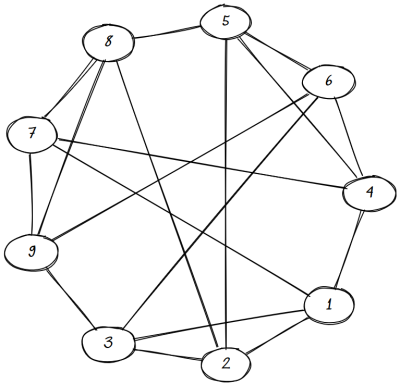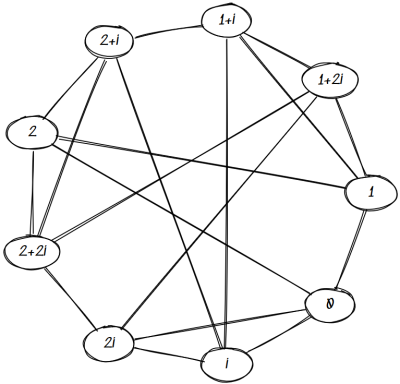# Rook graphs and Paley graphs

An m by n rook graph is formed by associating a node with each square of an m by n chess board and connecting two nodes with an edge if a rook can legally move from one to the other. That is, two nodes are connected if they are either in the same row or in the same column.

A Paley graph of order q is a graph with q nodes where q is a prime power and congruent to 1 mod 4. Label each node with an element of the finite field F with q elements and connect two nodes with an edge if the difference between their labels is a quadratic residue. That is, for nodes labeled a and b, connect these nodes if there exist an x in F such that ab = x².

We’ll look at a graph which is both a rook graph and a Paley graph: the 3 by 3 rook graph is the same as the Paley graph of order 9.

## Rook graph 3 x 3

Constructing the rook graph is easy. If we number our 3 by 3 chess board as follows

```    1 2 3
4 5 6
7 8 9
```

then we connect 1 to 2, 3, 4, and 7. We connect 2 to 1, 3, 5, and 8. Etc. This gives us the following graph.(The graph was made with Sketchviz introduced here.)

## Paley graph of order 9

They Paley graph is a little more complicated to construct. First we need to explain what a field with 9 elements is.

A field with 3 elements is just integers mod 3. We can think of the field with 9 elements as the analog of complex numbers: numbers of the form abi where a and b are integers mod 3. As with the complex numbers, i² = -1.

When we square each of the nine elements of our field, we get four distinct non-zero results: 1, 2, i, and 2i. For example, i is one of our squares because

(1 + 2i)(1 + 2i) = (1 – 4) + 4i = i

Here we used -3 = 0 mod 3 and 4 = 1 mod 3.

Since our squares are 1, 2, i, and 2i, that says 0 is connected to these four values. Then we connect 1 to 2, 0, 1 + i and 1 + 2i because each of these numbers minus 1 is a square. If we keep doing this for all nine nodes, we get the following graph.## Isomorphism

It’s clear that the two graphs above are relabelings of each other. We can see this explicitly by relabeling our chess board as follows:

```    0    i    2i
1  1+i  1+2i
2  2+i  2+2i
```

Moving horizontally adds i, which is a square, and moving vertically adds 1, which is a square. So the Paley connections are rook connections.

## Uniqueness

Are there more rook graphs that are isomorphic to Paley graphs? No, this is the only one.

Every pair of vertices in a rook graph that are not neighbors have two common. If the points with coordinates (a, b) and (c, d) are on different rows and columns, then both are connected to (a, d) and (c, b).

In a Paley graph of order q, every pair of nodes that aren’t neighbors have (q – 1)/4 common neighbors. For a Paley graph to be isomorphic to a rook graph, we must have (q – 1)/4 = 2, and so q = 9.

## 3 thoughts on “Rook graphs and Paley graphs”

1. Thanks. I’m fixing it now.

2. Nathan Hannon

Technically there’s one other case: the 1×2 rook graph is isomorphic to the Paley graph of order 2 (both consist of 2 nodes joined by an edge). This doesn’t contradict your counting argument because there are no pairs of non-adjacent nodes.

3. The rook graph shows 5 edges for node 8 and node 4, because node 8 and node 4 are show connected. I didn’t expect node 8 and 4 to be connected?